# The cube - simple

Calculate the surface of the cube measuring 15 centimeters.

S =  1350 cm2

### Step-by-step explanation: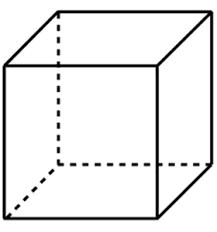Did you find an error or inaccuracy? Feel free to write us. Thank you!## Related math problems and questions:

• Cube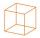Calculate the surface cube with edge 11 dm.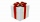How much wrapping paper is needed to wrap a cube-shaped gift with an edge measuring 15 cm?
• Net of a cube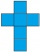We can fold this net to form a cube with a side length of 20 units. What is the surface area of the cube in square units?
• Cube surface areaThe surface of the cube was originally 216 centimeters square. The surface of the cube has shrunk from 216 to 54 centimeters sq. Calculate how much percent the edge of the cube has decreased.
• The cube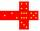The surface of the cube is 150 square centimeters. Calculate: a- the content of its walls b - the length of its edges
• A butterA butter cube with an edge 6.5 cm long is packed in a package with dimensions a = 28 cm, b = 15 cm. Calculate how many cm2 the package is larger than the surface of the cube.
• Cube 5The content area of one cube wall is 32 square centimeters. Determine the length of its edges, its surface and volume.
• Special cube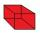Calculate the edge of cube, if its surface and its volume is numerically equal number.
• Two walls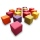Calculate the surface area of a cube in m2 if you know that the area of its two walls is 72 dm2.
• Cube surface areaThe Wall of the cube has a content area 99 cm square. What is the surface of the cube?
• Surface area of a cubeWhat is the surface area of a cube that has an edge of 3.5?
• Cube V2SThe volume of the cube is 27 dm cubic. Calculate the surface of the cube.
• The cubeThe cube has a surface area of 486 m ^ 2. Calculate its volume.
• Cube edgesThe sum of the lengths of the cube edges is 42 cm. Calculate the surface of the cube.
• Concrete pedestalThe carpenters made wooden mold on a concrete pedestal in the shape of a cube with an edge 2 meters long. What is the area in which the concrete touches wooden molds? (No lid or bottom)
• Cube 2How many times will increase if the surface area of the cube if we triple length of its edge?
• Cuboid - VabFind the surface of the cuboid when its volume is 52.8 cubic centimeters, and the length of its two edges is 2 centimeters and 6 centimeters.